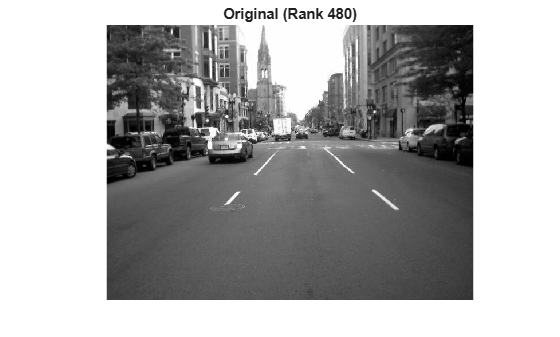# Image Compression with Low-Rank SVD

This example shows how to use `svdsketch` to compress an image. `svdsketch` uses a low-rank matrix approximation to preserve important features of the image, while filtering out less important features. As the tolerance used with `svdsketch` increases in magnitude, more features are filtered out, changing the level of detail in the image.

Load the image `street1.jpg`, which is a picture of a city street. The 3-D matrix that forms this image is `uint8`, so convert the image to a grayscale matrix. View the image with an annotation of the original matrix rank.

```A = imread('street1.jpg'); A = rgb2gray(A); imshow(A) title(['Original (',sprintf('Rank %d)',rank(double(A)))])```### Compress Image

Use `svdsketch` to calculate a low-rank matrix that approximates `A` within a tolerance of `1e-2`. Form the low-rank matrix by multiplying the SVD factors returned by `svdsketch`, convert the result to `uint8`, and view the resulting image.

```[U1,S1,V1] = svdsketch(double(A),1e-2); Anew1 = uint8(U1*S1*V1'); imshow(uint8(Anew1)) title(sprintf('Rank %d approximation',size(S1,1)))````svdsketch` produces a rank 288 approximation, which results in some minor graininess in some of the boundary lines of the image.

Now, compress the image a second time using a tolerance of `1e-1`. As the magnitude of the tolerance increases, the rank of the approximation produced by `svdsketch` generally decreases.

```[U2,S2,V2] = svdsketch(double(A),1e-1); Anew2 = uint8(U2*S2*V2'); imshow(Anew2) title(sprintf('Rank %d approximation',size(S2,1)))```This time, `svdsketch` produces a rank 48 approximation. Most of the major aspects of the image are still visible, but the additional compression increases the blurriness.

### Limit Subspace Size

`svdsketch` adaptively determines what rank to use for the matrix sketch based on the specified tolerance. However, you can use the `MaxSubspaceDimension` name-value pair to specify the maximum subspace size that should be used to form the matrix sketch. This option can produce matrices that do not satisfy the tolerance, since the subspace you specify might be too small. In these cases, `svdsketch` returns a matrix sketch with the maximum allowed subspace size.

Use `svdsketch` with a tolerance of `1e-1` and a maximum subspace size of 15. Specify a fourth output to return the relative approximation error.

`[U3,S3,V3,apxErr] = svdsketch(double(A),1e-1,'MaxSubspaceDimension',15);`

Compare the relative approximation error of the result with the specified tolerance. `apxErr` contains one element since `svdsketch` only needs one iteration to compute the answer.

`apxErr <= 1e-1`
```ans = logical 0 ```

The result indicates that the matrix sketch does not satisfy the specified tolerance.

View the heavily compressed rank 15 image.

```Anew3 = uint8(U3*S3*V3'); imshow(Anew3) title(sprintf('Rank %d approximation',size(S3,1)))```### Compare Results

Finally, view all of the images side-by-side for comparison.

```tiledlayout(2,2,'TileSpacing','Compact') nexttile imshow(A) title('Original') nexttile imshow(Anew1) title(sprintf('Rank %d approximation',size(S1,1))) nexttile imshow(Anew2) title(sprintf('Rank %d approximation',size(S2,1))) nexttile imshow(Anew3) title(sprintf('Rank %d approximation',size(S3,1)))```